• Shuffle
Toggle On
Toggle Off
• Alphabetize
Toggle On
Toggle Off
• Front First
Toggle On
Toggle Off
• Both Sides
Toggle On
Toggle Off
Toggle On
Toggle Off
Front

### How to study your flashcards.

Right/Left arrow keys: Navigate between flashcards.right arrow keyleft arrow key

Up/Down arrow keys: Flip the card between the front and back.down keyup key

H key: Show hint (3rd side).h key

A key: Read text to speech.a keyPlay buttonPlay buttonProgress

1/26

Click to flip

### 26 Cards in this Set

• Front
• Back
 Probability Theory why is it important explaining genetic events? • Independent Events • Chance of event 1 occurring is independent of event 2 • Product Law: multiply the probability of each event to determine probability of both events how do we calculate when two or more event occurr indpendently of one another but at the same time? Product Law: multiply the probability of each event to determine probability of both events give an example of the Product Law of their probability? Heads and Tails • P(Heads) on a single flip of the coin = 1/2 • P(Tails) on a single flip of the coin= 1/2 • eg. P(H)P(T) = 1/2 x 1/2 = 1/4 • P(H)P(H) = 1/2 x 1/2 = 1/4 • P(T)P(H) = 1/2 x 1/2 = 1/4 • P(T)P(T) = 1/2 x 1/2 = 1/4 • P(T)P(T)P(T) = 1/2 x 1/2 x 1/2 = 1/8 • 2 or more events happening simultaneously = product of their individual probabilities What is the Sum Law? • Outcome can be accomplished in more than one way • Sum the probabilities of each way of obtaining the outcome • eg. toss 2 pennies, what is the outcome of one head & one tail: PH xPT + PT x PH • 1/2 x 1/2 + 1/2 x 1/2 = 1/4 + 1/4 = 1/2 define what it meants independent assortment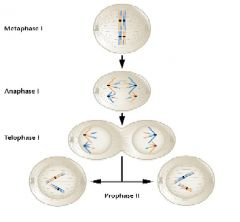independent behavior of each pair of homologous chromosomes during segregation in meisosis I. the random distribution of maternal and paternal homologs into gametes. ----------------------------- • 1st meiotic anaphase, homologs separate independently • Possible combinations = 2n • Humans: n = 23, therefore 223 = 8 x 106 • 8 million gametes possible just because of random assortment of homologous chromosomes What did Mendel’s experiments study? • Used common garden pea • Followed discrete traits • Kept detailed records • Determined units of inheritance exist &predicted their behavior during the formation of gametes • Basis of Mendelian or transmission genetics What did Mendel’s postulates? • Unit factors exist in pairs (alleles) • One unit factor is DOMINANT, the other is recessive • Paired unit factors segregate randomly what is reciprocal crosses? not sex dependt when mendel's monohybrid crosses what is particualate unit factors? factors serve as the basic units of heredity & are passed unchanged from generation to generation,determining various traits expressed by each individual plant. what is segregation monohybrid cross as determinant factor? during the formation of gametes, the paired unit factors separate, or segregate, randomly so that each gamete receives one or the other with equal likehood. four combination with equal fequency What happens in Monohybrid Cross?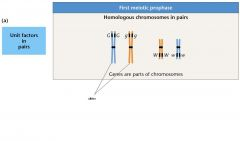• Trait transmission from one generation to the next • P1 = parental generation • F1 = first filial generation • F2 = second filial generation • Unit factors = genes filial: from the Latin filius “son” how does Sister chromatids of homologs segregate looks like?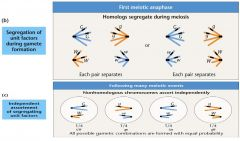ans Terms to Know • Phenotype = physical expression of a trait • Genotype = allelic makeup of a trait (DD,Dd) • Homozygote / homozygous = identical alleles (eg. DD or dd) • Heterozytoge / heterozygous = different alleles (eg. Dd) • Pure breeding lines (homozygous) alleles=different combination of alternative froms of single gene gene=unit factor representing of inheritance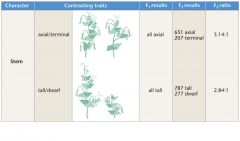Discuss some the result Mendel experiment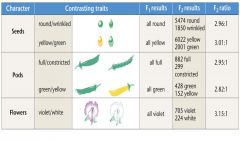seven pairs of contrasting traits of garden pea (Pisum Sativum). pollen derived from plants exhibiting one trait was used to fertilize the ova of plants exhibiting the other trait. .F1 generatiion, one of the two traits(domainant) was exhibiting by all plants. those trail in which each trait appeared unchanged generation after generation in self-fertilizing plants what led Mendel to deduce that unit factors exist in pairs? because there were two constracting traits for each character it would seem that two distinct factor must exist why does one of the two traits or phenotypes disappear in the F1 generation? observing F2, the recessive traits and its unit factor don't actually disappear in the F1; they are merely hidden or masked, only to reappear in one fourth of the F2 offspring. therefore, Mendel concluded that one unit factor for tall and one for dwarf were transmitted to each F1 individual, but because the tall factor or allele is dominant to the dwarf factor or allele, all F1 plants are tall. Why is punnet Square important?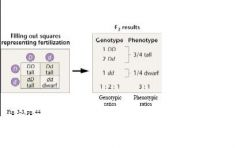the genotypes and phenotypes resulting from the recombination of gametes during fertilization can be easily visualized by constructing punnet square horizantal for male and verical for females or colums 3:1 phenotypic ratio 1:2:1 genotypic ratio may deriven from F2 generation How do you conduct a test cross?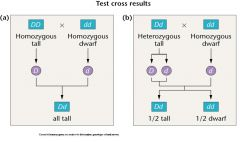it helps us distinguish the genotypes of plant expressing the dominant type What happens in Dihybrid cross? • Follow 2 pairs of contrasting traits • Independent genes • Independent events (can use product law) • Probability calculation (forked line method) What was Mendel's dihybrid cross revealed his four postulate?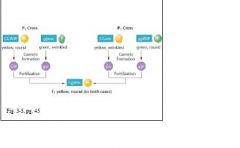Independent Assortment examined two characters simultaneously dihydrid cross Explain Independent Assortment?the chance of any plant having yellow or green seeds is not all all influenced by the chance that this plant will have round or wringle seed. two sets of traits as being inherited independently of each other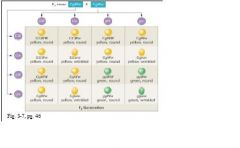dihybrid cross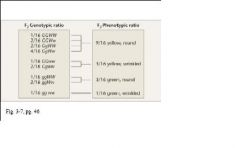result Fork line method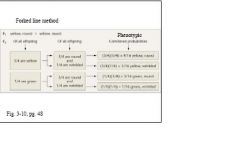result What is Test Cross- 2 Characters?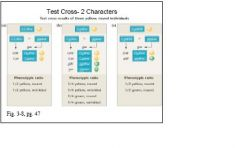result #’s of genotypes & phenotypes • First, determine # of heterozygous gene pairs in cross (n) • 2n = # of different gametes • 2n = # of different phenotypes • 3n = # of different genotypes Trihybrid Cross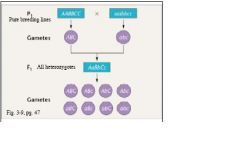• 3 factors • Punnett squares cumbersome • Use forked-line method for phenotypic calculations